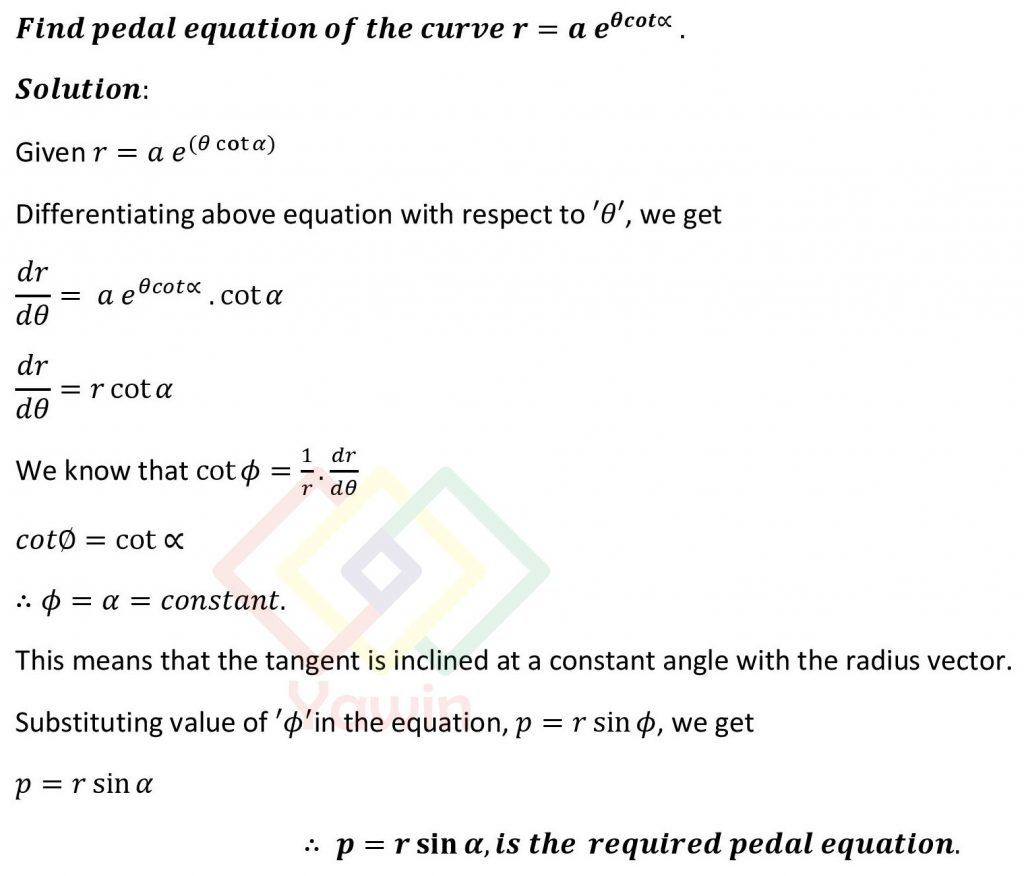Find pedal equation of the curve r=a e^ ((theta)cot(alpha)).

## Question## Question

Find pedal equation of the curve r=a e^ ((theta)cot(alpha)).

## Topic

Pedal equation of a polar curve